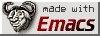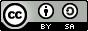... my hack.
```;;scad.el --- Major mode for editing SCAD files
;; Original author: Len Trigg <lenbok@gmail.com>
;; Maintainer: This hack is maintained by tca  <tca@diale.org>
;; Created:    20141107
;; Modified:   20141107
;; Version:    0.1
;;
;; This program is free software; you can redistribute it and/or modify
;; the Free Software Foundation; either version 2 of the License, or
;; (at your option) any later version.
;;
;; This program is distributed in the hope that it will be useful,
;; but WITHOUT ANY WARRANTY; without even the implied warranty of
;; MERCHANTABILITY or FITNESS FOR A PARTICULAR PURPOSE.  See the
;; GNU General Public License for more details.
;;
;; You should have received a copy of the GNU General Public License
;; along with this program; see the file COPYING.  If not, write to
;; the Free Software Foundation, Inc., 59 Temple Place - Suite 330,
;; Boston, MA 02111-1307, USA.
;;
;;; Commentary:
;;
;; This is a major-mode to implement the SCAD constructs and
;;; Code:

:type 'string)

'("return" "true" "false")
:type 'list

'("cos" "acos" "sin" "asin" "tan" "atan" "atan2"
"abs" "sign" "rands" "min" "max"
"round" "ceil" "floor"
"pow" "sqrt" "exp" "log" "ln"
"str"
"lookup" "version" "version_num" "len" "search"
"dxf_dim" "dxf_cross")
:type 'list

'("child" "children" "echo" "assign" "for" "intersection_for" "if"
"else" "cube" "sphere" "cylinder" "polyhedron" "square" "circle"
"polygon" "scale" "rotate" "translate" "mirror" "multmatrix" "union"
"difference" "intersection" "render" "color" "surface"
"dxf_linear_extrude" "linear_extrude" "dxf_rotate_extrude"
"rotate_extrude" "import_stl"
"import_off" "import_dxf" "import" "group"
"projection""minkowski" "glide" "subdiv" "hull" "resize")
:type 'list

'("+" "-" "*" "/" "%"
"&&" "||" "!"
"<" "<=" "==" "!=" ">" ">="
"?" ":" "=")
:type 'list

;; "C-c letter"  are reserved for users

(let ((map (make-sparse-keymap)))
(define-key map (kbd "C-c C-v") 'scad-open-current-buffer)
(define-key map (kbd "C-c C-c") 'scad-output-stl)

;; s for solid
(define-key map (kbd "C-c C-s c") 'scad-cylinder)
(define-key map (kbd "C-c C-s u") 'scad-cube)
(define-key map (kbd "C-c C-s y") 'scad-cylinder)
(define-key map (kbd "C-c C-s p") 'scad-polyhedron)

;; b for Boolean
(define-key map (kbd "C-c C-b u") 'scad-union)
(define-key map (kbd "C-c C-b d") 'scad-difference)
(define-key map (kbd "C-c C-b i") 'scad-intersection)

;; t for transformations
(define-key map (kbd "C-c C-t t") 'scad-translate)
(define-key map (kbd "C-c C-t r") 'scad-rotate)
(define-key map (kbd "C-c C-t s") 'scad-scale)
(define-key map (kbd "C-c C-t z") 'scad-resize)
(define-key map (kbd "C-c C-t m") 'scad-mirror)
(define-key map (kbd "C-c C-t x") 'scad-multmatrix)
(define-key map (kbd "C-c C-t c") 'scad-color)
(define-key map (kbd "C-c C-t h") 'scad-hull)
(define-key map (kbd "C-c C-t k") 'scad-minkowski)

;; s for syntax
(define-key map (kbd "C-c C-s m") 'scad-module)
(define-key map (kbd "C-c C-s f") 'scad-function)
(define-key map (kbd "C-c C-s i") 'scad-include)
(define-key map (kbd "C-c C-s u") 'scad-use)

;; m for math
;; Is this really needed;)

;; f for functions
(define-key map (kbd "C-c C-f l") 'scad-lookup)
(define-key map (kbd "C-c C-f r") 'scad-str)
(define-key map (kbd "C-c C-f c") 'scad-chr)
(define-key map (kbd "C-c C-f s") 'scad-search)
(define-key map (kbd "C-c C-f n") 'scad-version-num)
(define-key map (kbd "C-c C-f n") 'scad-norm )
(define-key map (kbd "C-c C-f c") 'scad-cross)
(define-key map (kbd "C-c C-f m") 'scad-parent-module)

;; o for others
(define-key map (kbd "C-c C-o r") 'scad-render)
(define-key map (kbd "C-c C-o f") 'scad-for)
(define-key map (kbd "C-c C-o t") 'scad-intersection-for)
(define-key map (kbd "C-c C-o i") 'scad-if)
(define-key map (kbd "C-c C-o a") 'scad-assign)
(define-key map (kbd "C-c C-o m") 'scad-import)
(define-key map (kbd "C-c C-o x") 'scad-linear-extrude)
(define-key map (kbd "C-c C-o o") 'scad-rotate-extrude)
(define-key map (kbd "C-c C-o s") 'scad-surface)
(define-key map (kbd "C-c C-o p") 'scad-projection)
(define-key map (kbd "C-c C-o c") 'scad-children)

;; p for on the plane
(define-key map (kbd "C-c C-p c") 'scad-circle)
(define-key map (kbd "C-c C-p s") 'scad-square)
(define-key map (kbd "C-c C-p p") 'scad-polygon)

(define-key map [return] 'newline-and-indent)
map)

(let ((st (make-syntax-table)))
;; support comment style: “// ...”
;; support comment style: “/* ... */”
(modify-syntax-entry ?\/ ". 124b" st)
(modify-syntax-entry ?\n "> b" st)
(modify-syntax-entry ?* ". 23" st)

;; Extra punctuation
(modify-syntax-entry ?+  "." st)
(modify-syntax-entry ?-  "." st)
(modify-syntax-entry ?%  "." st)
(modify-syntax-entry ?<  "." st)
(modify-syntax-entry ?>  "." st)
(modify-syntax-entry ?&  "." st)
(modify-syntax-entry ?:  "." st)
(modify-syntax-entry ?|  "." st)
(modify-syntax-entry ?=  "." st)
(modify-syntax-entry ?\;  "." st)

;; _ allowed in word (alternatively "_" as symbol constituent?)
(modify-syntax-entry ?_  "w" st) st)

`(
("\\(module\\|function\\)[ \t]+\\(\\sw+\\)" (1 'font-lock-keyword-face nil) (2 'font-lock-function-name-face nil t))
("\\(use\\|include\\)[ \t]*<\\([^>]+\\)>" (1 'font-lock-preprocessor-face nil) (2 'font-lock-type-face nil t))
("<\\(\\sw+\\)>" (1 'font-lock-builtin-face nil))
("\$\\(\\sw+\\)" (1 'font-lock-builtin-face nil))
;(,scad-operators-regexp .  font-lock-operator-face) ;; This actually looks pretty ugly
;("\\(\\<\\S +\\>\\)\\s *(" 1 font-lock-function-name-face t) ;; Seems to override other stuff (e.g. in comments and builtins)
)

"A major mode for editing SCAD files."
;; set comment styles for scad mode
(set (make-local-variable 'comment-start) "//")
(set (make-local-variable 'comment-end) "")
(set (make-local-variable 'block-comment-start) "/*")
(set (make-local-variable 'block-comment-end) "*/"))

"Makes a hidden scad-mode buffer containing all the scad keywords, so dabbrev expansion just works."
(insert "module function use include")  ; Explicitly add these -- they're not in the below vars

"Indent current line of SCAD code."
(interactive)
(let ((savep (> (current-column) (current-indentation)))
(if savep
(save-excursion (indent-line-to indent))
(indent-line-to indent))))

"Return the column to which the current line should be indented."
(save-excursion
(let ((pos (point)))
(beginning-of-line)
(if (not (search-backward-regexp "[^\n\t\r ]" 1 0))
0
(progn
(+ (current-indentation) (* standard-indent (scad-count-scope-depth (point) pos))))))))

(beginning-of-line)
(forward-to-indentation 0)
(if (looking-at "\\s)")
(forward-char)
(beginning-of-line)))

"Return face descriptor for char at point."
(plist-get (text-properties-at pos) 'face))

"Return difference between open and close scope delimeters."
(save-excursion
(goto-char rstart)
(let ((open-count 0)
(close-count 0)
opoint)
(while (and (< (point) rend)
(progn (setq opoint (point))
(re-search-forward "\\s)\\|\\s(" rend t)))
(if (= opoint (point))
(forward-char 1)
(cond
;; Don't count if in string or comment.
((looking-back "\\s)")
(setq close-count (+ close-count 1)))
((looking-back "\\s(")
(setq open-count (+ open-count 1)))
)))
(- open-count close-count))))

(interactive)
(call-process scad-command nil 0 nil (buffer-file-name)))

(interactive)
(async-shell-command (concat  scad-command " -o " (buffer-file-name) ".stl "
(buffer-file-name))))

;; Skeleton for easy editing
;; 3D
"Insert sphere."
"Sphere:"
"sphere:(" str ");")

"Insert cube."
"Cube:"
"cube:(" str ");")

"Insert cylinder."
"Cylinder:"
"cylinder(" str ");")

"Insert polyhedron."
"Polyhedron:"
"polyhedron(" str ");")

;; 2D

"Insert square."
"Square:"
"square(" str ");")

"Insert square ."
"Square:"
"square(" str ");")

"Insert polygon."
"Polygon:"
"Polygon(" str ");")

;; Transformations

"Insert translate."
"translate:"
"translate(" str "){"_"}")

"Insert rotate."
"rotate:"
"rotate(" str "){"_"}")

"Insert scale."
"Scale:"
"scale(" str "){"_"}")

"Insert resize."
"Resize:"
"resize(" str "){"_"}")

"Insert mirror."
"Mirror:"
"mirror(" str "){"_"}")

"Insert multmatrix."
"Multmatrix:"
"multmatrix:(" str "){"_"}")

"Insert color."
"Color:"
"color(" str "){"_"}")

"Insert hull."
"Hull:"
"hull(" str "){"_"}")

"Insert minkowski."
"Minkowski:"
"minkowski(){"_"}")

;; Boolean

"Insert union."
"Union:"
"union(){}")

"Insert difference."
"Difference:"
"difference(){"_"}")

"Insert intersection."
"Intersection:"
"intersection(){"_"}")

;; Syntax

"Insert module."
"Module:"
"module " str "("_"){}")

"Insert function."
"Function:"
"function " str "("_"){}")

"Insert include."
"Include:"

"Insert use."
"Use:"

;; Other

"Insert render."
"Render:"
"render(){"_"}")

"Insert translate."
"translate:"
"translate(" str "){"_"}")

"Insert rotate."
"rotate:"
"rotate(" str "){"_"}")

"Insert scale."
"Scale:"
"scale(" str "){"_"}")

"Insert resize."
"Resize:"
"resize(" str "){"_"}")

"Insert mirror."
"Mirror:"
"mirror(" str "){"_"}")

"Insert multmatrix."
"Multmatrix:"
"multmatrix:(" str "){"_"}")

"Insert color."
"Color:"
"color(" str "){"_"}")

"Insert hull."
"Hull:"
"hull(" str "){"_"}")

"Insert minkowski."
"Minkowski:"
"minkowski(){"_"}")

;; Boolean

"Insert union."
"Union:"
"union(){}")

"Insert difference."
"Difference:"
"difference(){"_"}")

"Insert intersection."
"Intersection:"
"intersection(){"_"}")

;; Syntax

"Insert module."
"Module:"
"module " str "("_"){}")

"Insert function."
"Function:"
"function " str "("_"){}")

"Insert include."
"Include:"

"Insert use."
"Use:"

;; Other

"Insert render."
"Render:"
"render(){"_"}")

"Insert for."
"For:"
"for  (i=" str ")" "{" _ "}")

;;   "Insert ."
;;   ":"
;;   "(" str ");")

```

Enjoy.